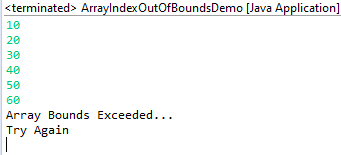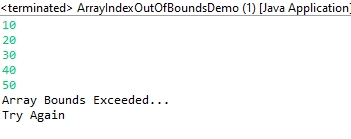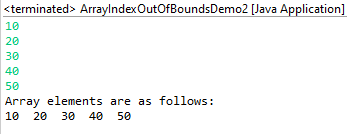# Java Program to Check Array Bounds while Inputing Elements into the Array

• Last Updated : 23 Jun, 2021

Concept: Arrays are static data structures and do not grow automatically with an increasing number of elements. With arrays, it is important to specify the array size at the time of the declaration. In Java, when we try to access an array index beyond the range of the array it throws an ArrayIndexOutOfBounds Exception. An exception is an obstruction to the normal program execution. Java has try-catch-finally blocks for efficient Exception Handling. ArrayIndexOutOfBoundsException is a runtime exception and must be handled carefully to prevent abrupt termination of the program.

Approaches:

1. Using try-catch block where input is taken beyond the array index range
2. Using try-catch block where input is taken within the array index range
3. Using constraints over user input

First Approach

In the first approach, an array of size = 5 is declared. The input is taken within a try block and the loop is executed 6 times. Since the array size is 5, after the 6th input an ArrayIndexOutOfBoundsException is thrown. The exception is handled by the catch block. The code to handle the exception is placed within the catch block. In this example, we notify the user that an exception has occurred and the input exceeds the array range.

Implementation: In all approaches variable named ‘i’ is used of integer data-type been taken for consideration.

## Java

 `// Importing generic Classes/Files``import` `java.util.*;` `public` `class` `GFG {` `    ``// Main driver method``    ``public` `static` `void` `main(String args[])``        ``throws` `ArrayIndexOutOfBoundsException``    ``{` `        ``// Taking input from user``        ``Scanner s = ``new` `Scanner(System.in);` `        ``// Storing user input elements in an array``        ``int` `arr[] = ``new` `int``[``5``];` `        ``// Try block to check exception``        ``try` `{``            ``// Forcefully iteration loop no of times``            ``// these no of times > array size``            ``for` `(``int` `i = ``0``; i < ``6``; i++) {` `                ``// Storing elements through nextInt()``                ``arr[i] = s.nextInt();``            ``}``        ``}``        ``catch` `(ArrayIndexOutOfBoundsException e) {``            ``// Print message when any exception occurs``            ``System.out.println(``                ``"Array Bounds Exceeded...\nTry Again"``);``        ``}``    ``}``}`

Output:Second Approach

In the second approach, we declare an array of size = 5. The input is taken within a while loop inside a try block. The value of i is checked against the size of the array at every iteration. The value of ‘i’ is initiated with 0 and can take input up to index 4. As soon as the value of ‘i’ reaches 5 an exception is thrown. This exception is handled by the catch block. This method is similar to the first one, however, in this approach, no input is taken beyond the array index range which was not the case in the first approach.

Implementation:

## Java

 `// Importing generic Classes/Files``import` `java.util.*;` `public` `class` `GFG {` `    ``// Main driver method``    ``public` `static` `void` `main(String args[])``        ``throws` `ArrayIndexOutOfBoundsException``    ``{` `        ``// Taking input from user``        ``Scanner s = ``new` `Scanner(System.in);``        ``// Storing elements as array``        ``int` `arr[] = ``new` `int``[``5``];` `        ``/ variable created and initialized with ``0` `int` `i = ``0``;` `        ``// try block to check exception``        ``try` `{` `            ``// Condition check``            ``while` `(``true``) {``                ``if` `(i == ``5``)` `                    ``// Statement responsible for exception``                    ``throw` `new` `ArrayIndexOutOfBoundsException();``                ``arr[i++] = s.nextInt();``            ``}``        ``}` `        ``// Catch block to handle exception``        ``catch` `(ArrayIndexOutOfBoundsException e) {` `            ``// Message printed when exception occurs``            ``System.out.println(``                ``"Array Bounds Exceeded...\nTry Again"``);``        ``}``    ``}``}`

Output:Third Approach

In this approach we do not use the concept of exception handling rather we limit the input using loops. It is an easier and convenient method of checking array bounds while taking inputs from the user.

Implementation:

## Java

 `// Importing Classes/Files``import` `java.util.*;` `public` `class` `GFG {` `    ``// Main driver code``    ``public` `static` `void` `main(String args[])``    ``{` `        ``// Taking user input through scanner``        ``Scanner s = ``new` `Scanner(System.in);` `        ``// Creating array to store elements``        ``int` `arr[] = ``new` `int``[``5``];` `        ``// creating and initializing variable with 0``        ``int` `i = ``0``;` `        ``// Condition check``        ``while` `(i < ``5``) {` `            ``// Storing user defined elements in array``            ``arr[i++] = s.nextInt();``        ``}``        ``System.out.println(``            ``"Array elements are as follows: "``);` `        ``// Iteration over elements``        ``for` `(``int` `j = ``0``; j < ``5``; j++)``            ``System.out.print(arr[j] + ``"  "``);``    ``}``}`

Output:My Personal Notes arrow_drop_up